# Advanced Function for Calculating % Against Column

Joshua Baron shared this question ago

Hi there, I have created a report that shows Property Loss by Year/Quarter. As you can see from the screen grabs, I was able to successfully calculate the % property loss by using the "Percentage Against Column" advanced function. I chose the property loss amount against the property value column. This works perfectly except when I add subtotals (so I am summing by year) I can't figure out how make this column show the summed value of property loss as a % of the summed value of the property value. Any help would be appreciated - thanks!

Josh1

Hi Joshua,

Thanks for reaching out. Unless I'm misunderstanding your question, if you're trying to sum the values of the percentage values you'd just click the arrow next to the field you used the Advanced Function on, click totals, then Sum: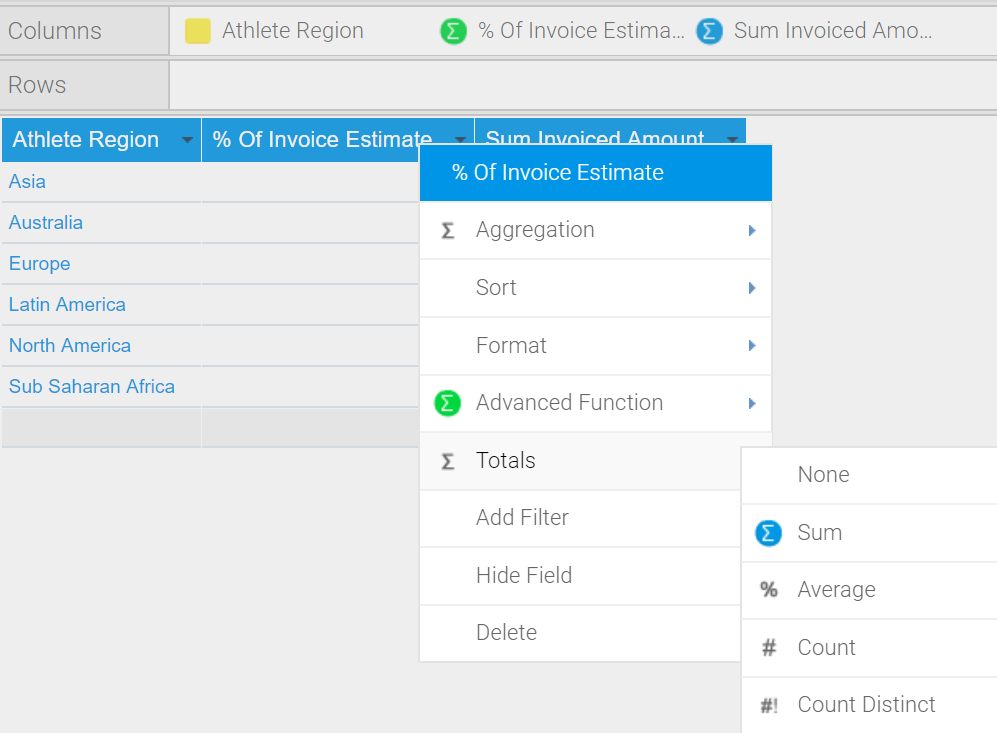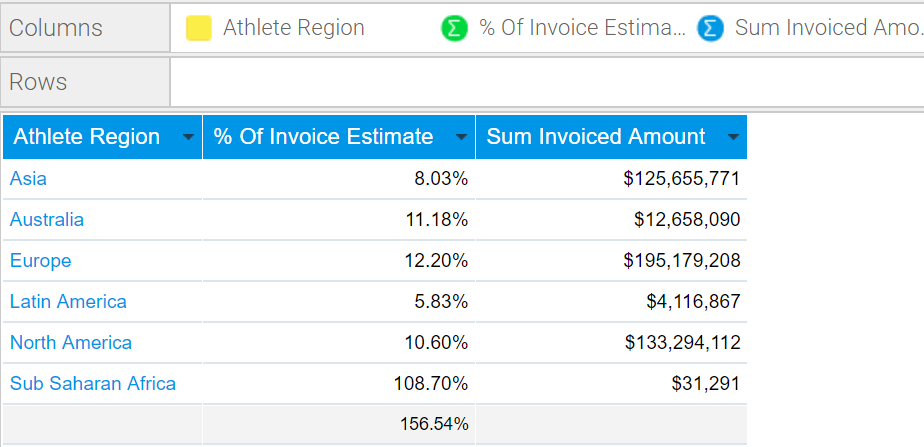Is this what you're looking for?

Regards,

Mike1

Thanks for the response. The issue is that I don't want to sum the percentages - I want the percentage to show up of the subtotals. To illustrate this point better, if you look at the second attachment you will see that I have circled in red the field that I am looking to calculate. The total of property loss for 2014 was \$2,225,050; the total of property value for 2014 was \$114,218,601. This should lead to \$2,225,050/\$114,218,601 = 1.9%. There is no way (summing, average, etc.) that I can see to get to that value. Hopefully that clarifies what I am looking for.

Thanks!1

Hi Joshua,

Thanks for your reply. In this case then, instead of using the % Against Column Advanced Function, I'd instead recommend creating a Calculated Field, then applying a Calculated Total to that.

As an example, I've created a Calculated Field with a formula of

SUM (Invoice Estimate) / SUM (Invoiced Amount) * 100.00 (to make it a percentage value)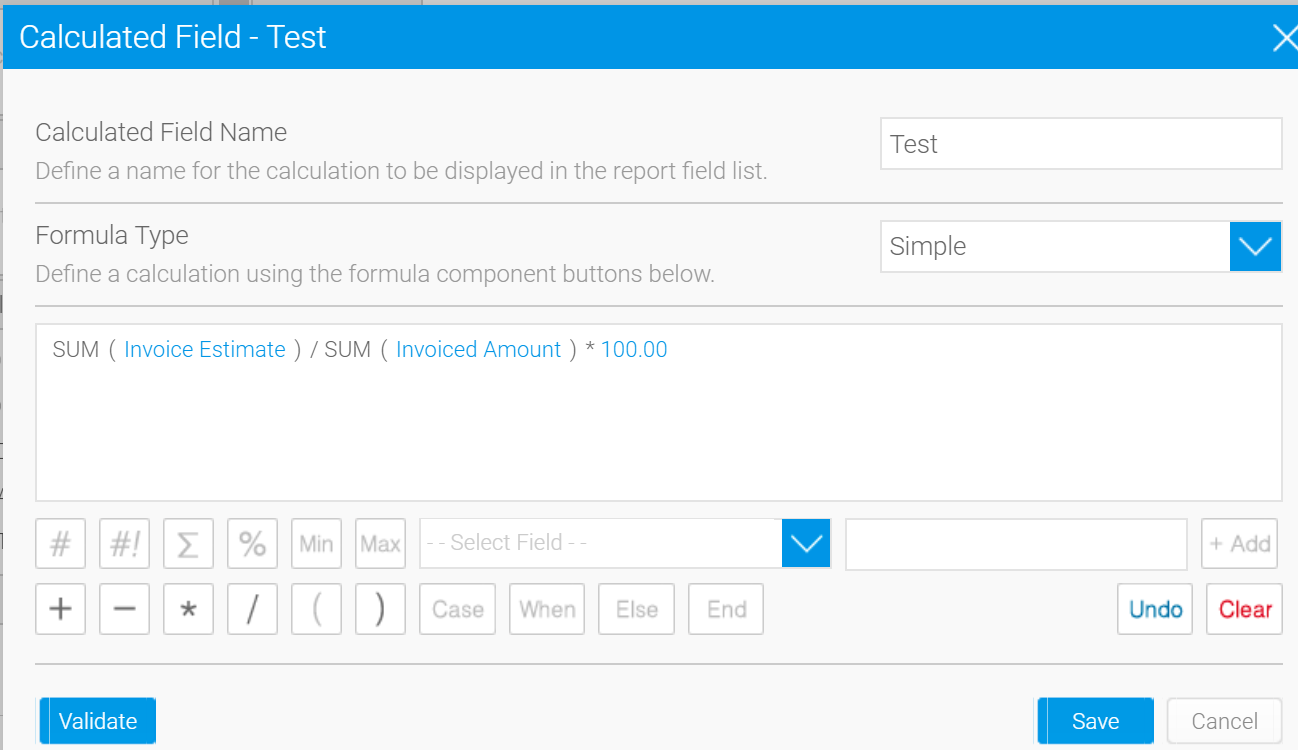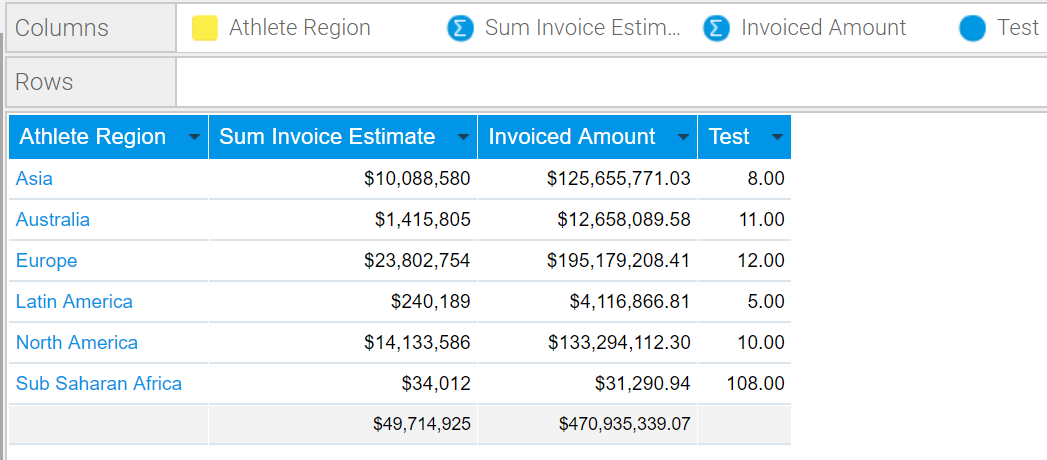Note that calculations are driver-dependent, so with our embedded HSQL database as you can see above, the row values are rounded in an unexpected way. All works as anticipated using MySQL and most standard RDBMS' though, as a side note, so it should work for you as well: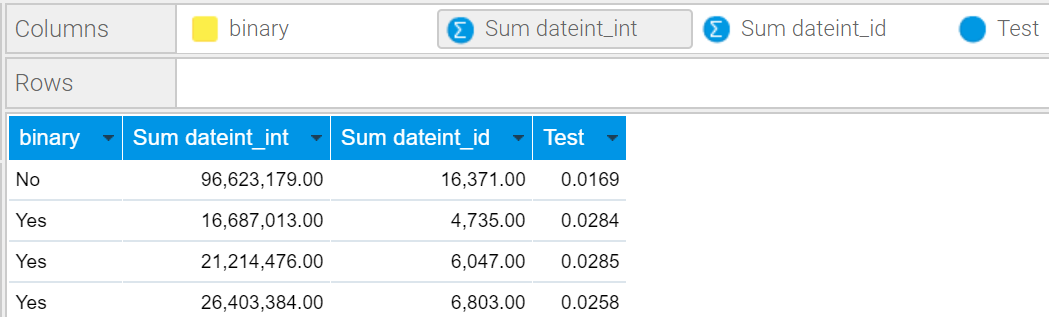Anyways, after scripting the Calculated Field and using Total > SUM on your other fields, you can now click the arrow next to the newly created Calculated Field > Totals > Calculated Total and you'll see the result you're looking for: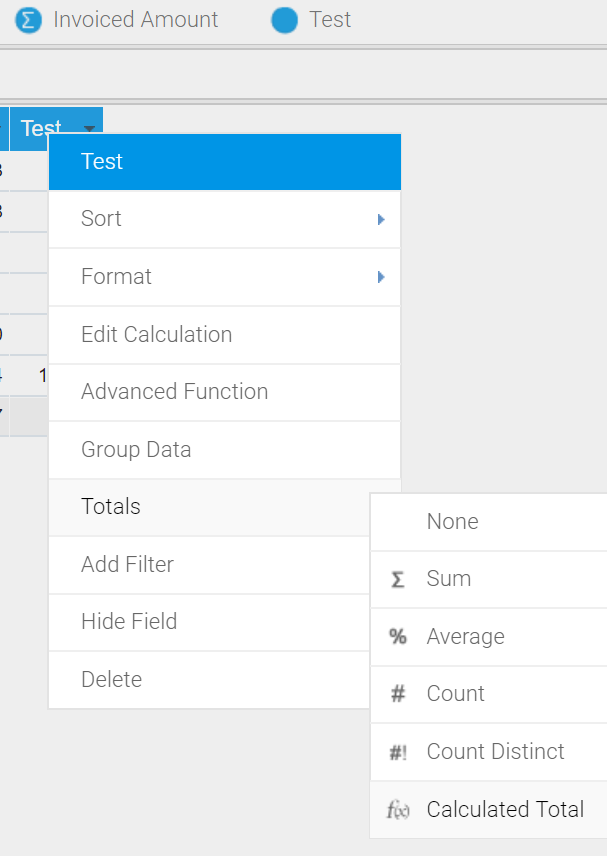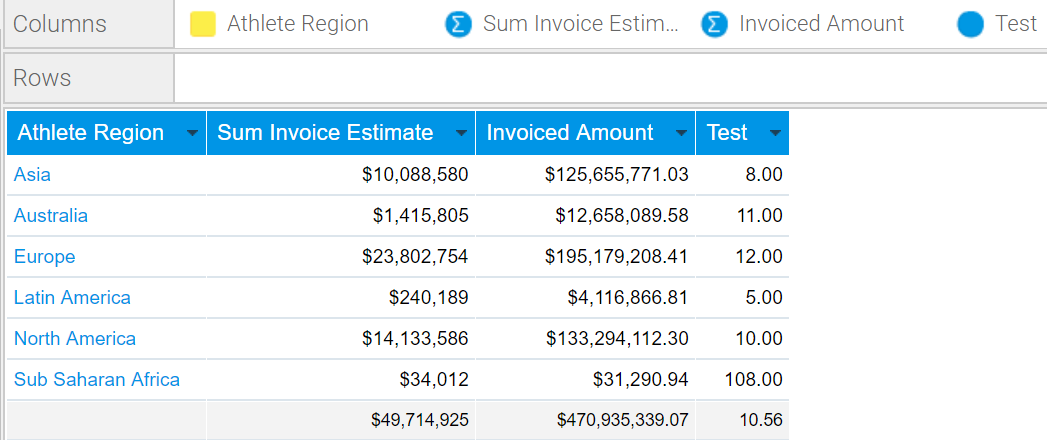If you divide 49714925 by 470935339.07 you'll see 10.56 is the correct result.

Please let me know how this goes.

Regards,

Mike1

That worked perfectly!! Thanks so much - I have a new question but I will post as a new thread :-)

Josh1

Hi Joshua,

Great! Happy to hear it. I'll close this case out then. Thanks for keeping issues separate.

Regards,

Mike